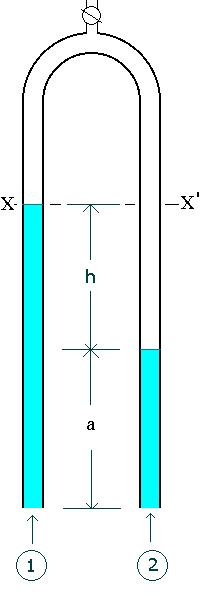### Inverted U - tube Manometer

Home -> Lecture Notes -> Fluid Mechanics -> Unit-IInverted U-tube manometer is used for measuring pressure differences in liquids. The space above the liquid in the manometer is filled with air which can be admitted or expelled through the tap on the top, in order to adjust the level of the liquid in the manometer.

Equating the pressure at the level XX'(pressure at the same level in a continuous body of static fluid is equal),

For the left hand side:

Px = P1 - rg(h+a)

For the right hand side:

Px' = P2 - (rga + rmgh)

Since Px = Px'

P1 - rg(h+a) = P2 - (rga + rmgh)

P1 - P2 = (r - rm)gh

If the manometric fluid is choosen in such a way that rm << r then,

P1 - P2 = rgh.

For inverted U - tube manometer the manometric fluid is usually air.